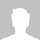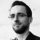Jedox Ideas

Let us know how we can make Jedox even better!

2
Workspace Jedox Platform
Categories
Created by Guest
Created on Jun 2, 2020

# Rules - Select NOT this target area

Currently the rules are pointed directly to a point in which it is calculated

Like this where the rule is calculated for all Month_Inv, but then ignores the "~" using if.

``````['Cost Driver':'LB_AREA_BBR', 'Investment Measure':'Amount', 'Version':{'Scenario_Base'}] = B:
IF(
!'Month_Inv' != "~"
,['Cost Driver':'PaymentScenario', 'Investment Measure':'Amount']
, continue()
)``````

What if we could write that on the left side of the expression?

``````['Month_Inv':{!'~'}, 'Cost Driver':'LB_AREA_BBR', 'Investment Measure':'Amount', 'Version':{'Scenario_Base'}] = B:
['Cost Driver':'PaymentScenario', 'Investment Measure':'Amount']
``````

I just invented some arbitrary syntax for the example, where "'Month_Inv':{!'~'}" is like NOT "~". Hence, being calculated for all Month_Inv that is not "~".

Being able to deselect here, could help avoid some rule templates, just to keep down the complexity of rules.

•Guest
Mar 4, 2022

Now there is also the possibility to use subsets in rules, which is a simpler alternative to rule templates.

•Guest
Sep 11, 2020

yes, but that again is a bit "klunky", as then I have another rule that has to run at a specific point to work, and the transparency is not great.

But thanks for the suggestion.... I still find the syntax thing more elegant, and optimizations can be done in the compiler to gain the most from the Jedox engine(s).

•